# Wieferich prime

This article defines a property that can be evaluated for a prime number. In other words, every prime number either satisfies this property or does not satisfy this property.
View other properties of prime numbers | View other properties of natural numbers

## Definition

A Wieferich prime is an odd primesuch that: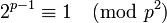.

In particular,is not a primitive root modulo the square of a Wieferich prime.

## Occurrence

### Initial examples

Currently, there are only two known Wieferich primes: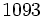and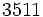.

### Infinitude conjecture

Further information: Finitude conjecture for Wieferich primes, Infinitude conjecture for Wieferich primes

It has been conjectured by some that there are only finitely many Wieferich primes.

It has also been conjectured that there are infinitely many Wieferich primes. Further, it is conjectured that the number of Wieferich primes less thanis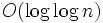. This explains the slow growth rate of Wieferich primes.

### Infinitude of complement

It is conjectured that there are infinitely many non-Wieferich primes (note that the truth of this conjecture would say nothing about the finitude or infinitude of the Wieferich primes).

The Erdos conjecture on non-existence of three consecutive powerful numbers implies the infinitude of non-Wieferich primes.

Also, the abc conjecture implies a logarithmic lower bound on the number of non-Wieferich primes less than: in other words, there exists a positive constant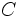such that if the abc conjecture is true, the number of non-Wieferich primes less than or equal tois bounded from below by.